Powered by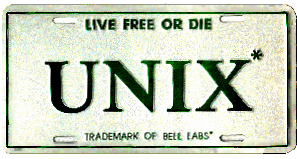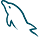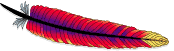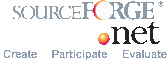© January 1, 2014 Lorentz JÄNTSCHI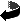Up
This program requires molecules stored as HyperChem files ('.hin').
The files should have the partial charges calculated.
The program produces a large table containing molecular descriptors computed on (Unsymmetrical) Szeged Sets, an extension of corresponding Szeged Matrices, collecting vertices in place of the numbers.
The table of descriptors lists the members of the Szeged Matrix Property Indices (SMPI) family of molecular descriptors, a new family of molecular descriptors created at same time with the current interface.
The genetic code of this family of molecular descriptors is given below and a descriptor name encodes in reversed order the values for the genes:
 Gene Genome Legend AP A B C D E F G AP=A: Atomic mass (a.u.), as sum of; AP=B: Atomic number (Z), as harmonic sum of; AP=C: Cardinality (=1), as sum of; AP=D: Solid state density (kg/m3), as harmonic mean of; AP=E: Electronegativity (Revised Pauling), as geometrical mean of; AP=F: First ionization energy (kJ/mol), as average of; AP=G: Melting point temperature (K), as Euler (PM(p), p=2) mean of; DM=T: Topological distance (bonds); DM=G: Geometrical distance (Ĺ); DM=U: Weighted topological distance (reversed bond order); ID=E: Ei,j=Pi,j*Di,j; ID=U: Ui,j=Pi,j/Di,j; ID=D: Di,j=1*Di,j; ID=P: Pi,j=Pi,j*1; MO=m: min; MO=M: max; MO=I: half-sum(Mi,j); MO=J: half-sum(Mi,j*Mj,i); MO=E: half-sum(Mi,j*Adi,j); MO=F: half-sum(Mi,j*Mj,i*Adi,j)LO=I: I(x)=x; LO=R: R(x)=1/x; LO=L: L(x)=Ln(x) DM T G U ID E U D P MO m M I J E F LO I R L
This free to use (very simple and very fast) software is intended to demonstrate the utility of using families of molecular descriptors.
The algorithmic complexity in this implementation do not overpass O(N4), where N is the number of heavy (<>'H') atoms.

 Data example